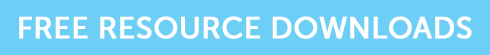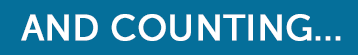Lies, Damned Lies and Statistics

# Lies, Damned Lies and Statistics

If you are studying for CII R02, J10 or AF4 exams then you need to get to grips with some statistical concepts. Our aim in this article is to set out in hopefully simple fashion how the use of statistics can help us to understand the level of risk inherent in an investment and how that relates to investment return.Risk is traditionally understood to be measured by volatility – the rate at which the price of an investment moves up and down. High volatility = price moves up and down rapidly over short time periods. Low volatility = price changes very slowly over a long time period.

The most common statistical measure of risk / volatility is Standard Deviation (sigma /  σ ) – a standard statistical measure not only relevant to investments. It’s an acceptable measure of risk if the probability distribution of returns is a normal distribution – i.e. where the distribution of expected return is spread symmetrically around the mean (average) and the graph of the distribution is a bell shaped curve, and investment theory assumes that this is the case.

A typical question on standard deviation is where you are given the figures for the mean annual return of an investment or portfolio over a number of years, and the figure for standard deviation (you are only likely to be asked to calculate the standard deviation in the AF4 exam). The numbers that you do need to remember then are :

68 – roughly the % of returns that will fall between the mean return plus and minus one sigma

95 – roughly the % of returns that will fall between the mean return plus and minus two sigma

It’s not magic, it’s maths – believe you me, just remember those two numbers and you won’t go far wrong.

Looking at an example to show how this works:

A portfolio has a mean return of 8% and a standard deviation of 2.5%. You can expect:

–          68% of future returns to fall within the range 5.5% to 10.5%

–          95% of future returns to fall within the range 3% to 13%

–          Conversely you can expect future returns below 3% and over 13% to occur only 5% of the time

Investment theory assumes that investors are logical and risk averse, and where two investments have the same expected return but different standard deviations, the rational investor will choose the investment with the lower standard deviation hence the lower level of risk.

Standard Deviation is the key measure of risk and it’s used in the calculation of other useful numbers which help us to understand risk and return, particularly alpha and beta.  And remember those numbers!

68 – Mexico City Olympic Games took place, Hey Jude gets to No 1 in the charts

95 – Windows 95 introduced! Not a very good mnemonic but 1995 was a particularly boring year!